# Linear Programming Model Formulation and Graphical Solution Chapter

• Slides: 26
Download presentation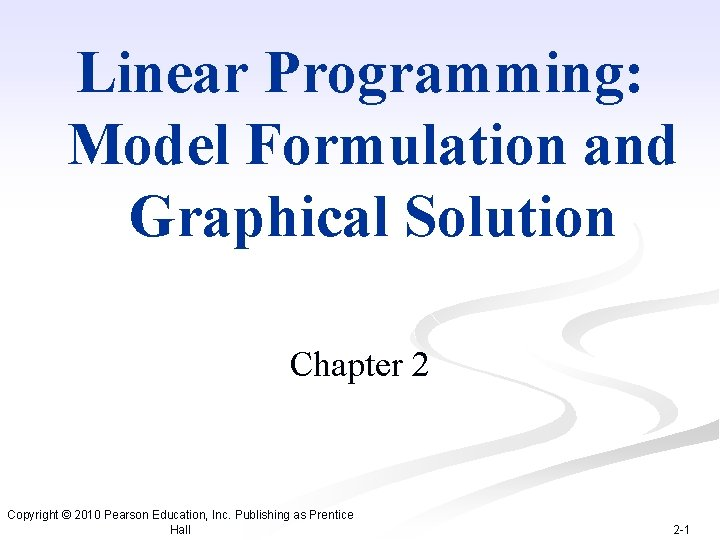Linear Programming: Model Formulation and Graphical Solution Chapter 2 Copyright © 2010 Pearson Education, Inc. Publishing as Prentice Hall 2 -1Chapter Topics n Model Formulation n A Maximization Model Example n Graphical Solutions of Linear Programming Models n A Minimization Model Example n Irregular Types of Linear Programming Models n Characteristics of Linear Programming Problems Copyright © 2010 Pearson Education, Inc. Publishing as Prentice Hall 2 -2Linear Programming: An Overview n Objectives of business decisions frequently involve maximizing profit or minimizing costs. n Linear programming uses linear algebraic relationships to represent a firm’s decisions, given a business objective, and resource constraints. n Steps in application: 1. Identify problem as solvable by linear programming. 2. Formulate a mathematical model of the unstructured problem. 3. Solve the model. 4. Implementation Copyright © 2010 Pearson Education, Inc. Publishing as Prentice Hall 2 -3Model Components n Decision variables - mathematical symbols representing levels of activity of a firm. n Objective function - a linear mathematical relationship describing an objective of the firm, in terms of decision variables - this function is to be maximized or minimized. n Constraints – requirements or restrictions placed on the firm by the operating environment, stated in linear relationships of the decision variables. n Parameters - numerical coefficients and constants used in the objective function and constraints. Copyright © 2010 Pearson Education, Inc. Publishing as Prentice Hall 2 -4Summary of Model Formulation Steps Step 1 : Clearly define the decision variables Step 2 : Construct the objective function Step 3 : Formulate the constraints Copyright © 2010 Pearson Education, Inc. Publishing as Prentice Hall 2 -5LP Model Formulation A Maximization Example (1 of 4) n Product mix problem - Beaver Creek Pottery Company n How many bowls and mugs should be produced to maximize profits given labor and materials constraints? n Product resource requirements and unit profit: Resource Requirements Labor (Hr. /Unit) Clay (Lb. /Unit) Profit (\$/Unit) Bowl 1 4 40 Mug 2 3 50 Product Copyright © 2010 Pearson Education, Inc. Publishing as Prentice Hall 2 -6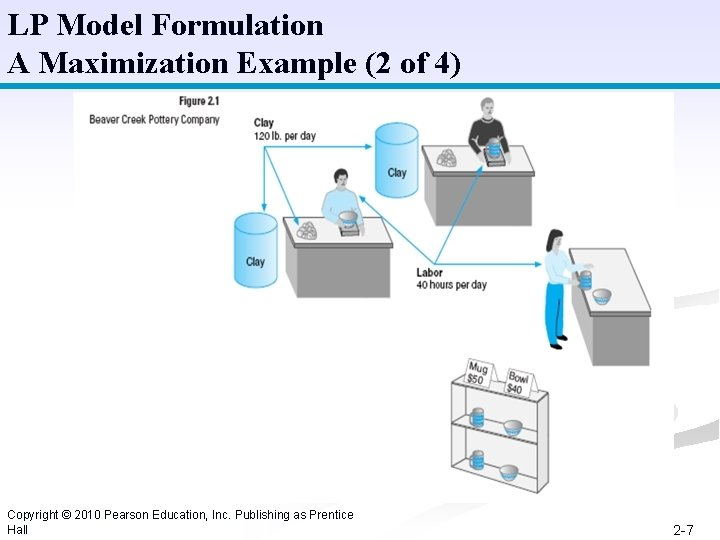LP Model Formulation A Maximization Example (2 of 4) Copyright © 2010 Pearson Education, Inc. Publishing as Prentice Hall 2 -7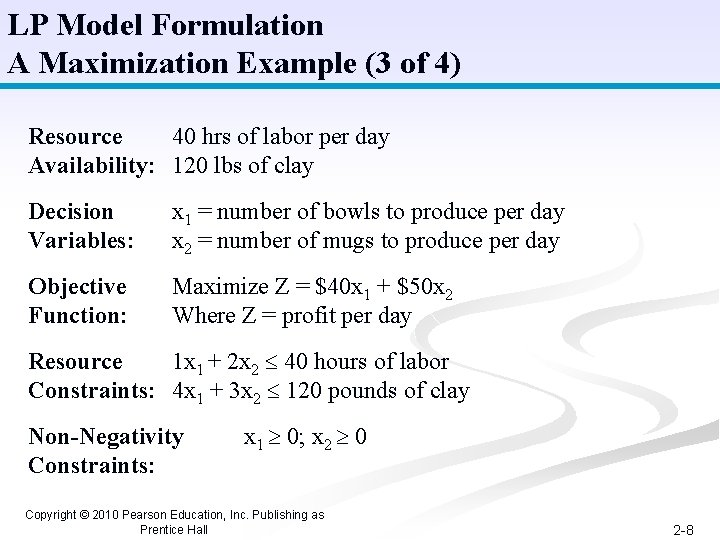LP Model Formulation A Maximization Example (3 of 4) Resource 40 hrs of labor per day Availability: 120 lbs of clay Decision Variables: x 1 = number of bowls to produce per day x 2 = number of mugs to produce per day Objective Function: Maximize Z = \$40 x 1 + \$50 x 2 Where Z = profit per day Resource 1 x 1 + 2 x 2 40 hours of labor Constraints: 4 x 1 + 3 x 2 120 pounds of clay Non-Negativity Constraints: x 1 0; x 2 0 Copyright © 2010 Pearson Education, Inc. Publishing as Prentice Hall 2 -8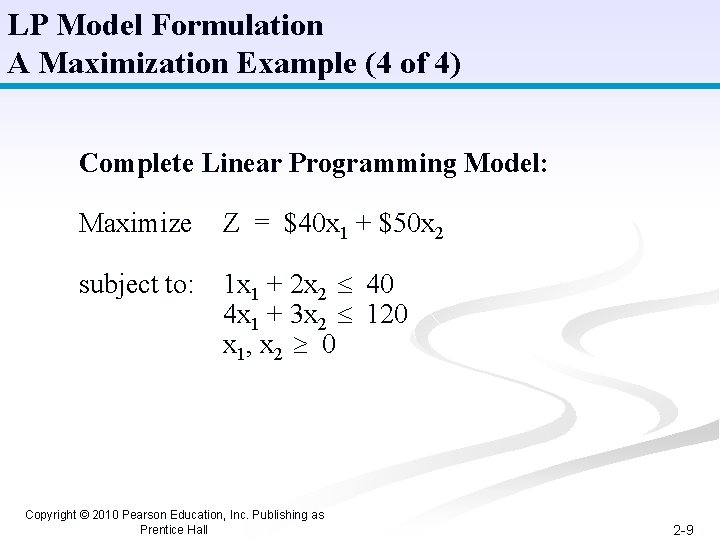LP Model Formulation A Maximization Example (4 of 4) Complete Linear Programming Model: Maximize Z = \$40 x 1 + \$50 x 2 subject to: 1 x 1 + 2 x 2 40 4 x 1 + 3 x 2 120 x 1, x 2 0 Copyright © 2010 Pearson Education, Inc. Publishing as Prentice Hall 2 -9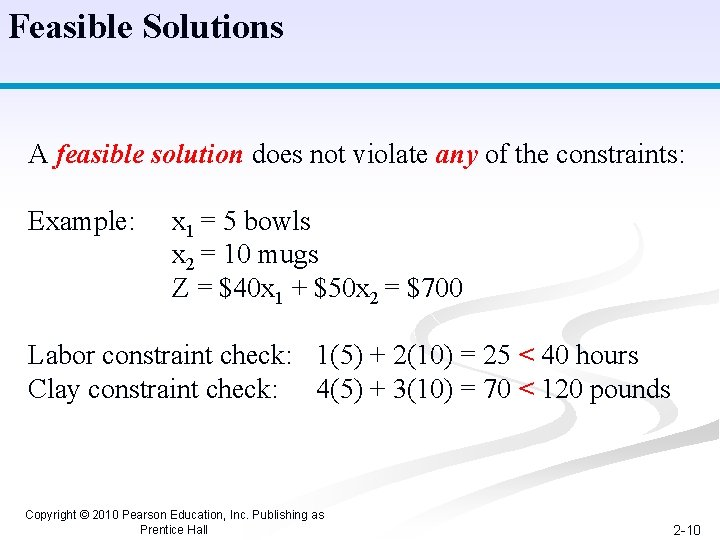Feasible Solutions A feasible solution does not violate any of the constraints: Example: x 1 = 5 bowls x 2 = 10 mugs Z = \$40 x 1 + \$50 x 2 = \$700 Labor constraint check: 1(5) + 2(10) = 25 < 40 hours Clay constraint check: 4(5) + 3(10) = 70 < 120 pounds Copyright © 2010 Pearson Education, Inc. Publishing as Prentice Hall 2 -10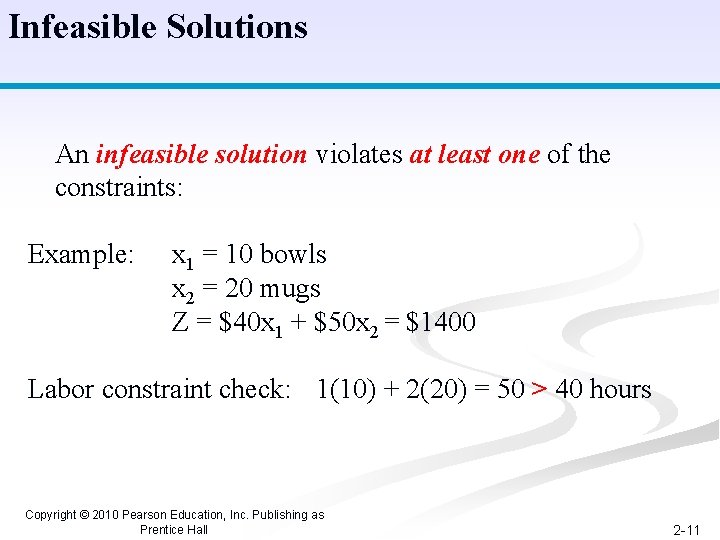Infeasible Solutions An infeasible solution violates at least one of the constraints: Example: x 1 = 10 bowls x 2 = 20 mugs Z = \$40 x 1 + \$50 x 2 = \$1400 Labor constraint check: 1(10) + 2(20) = 50 > 40 hours Copyright © 2010 Pearson Education, Inc. Publishing as Prentice Hall 2 -11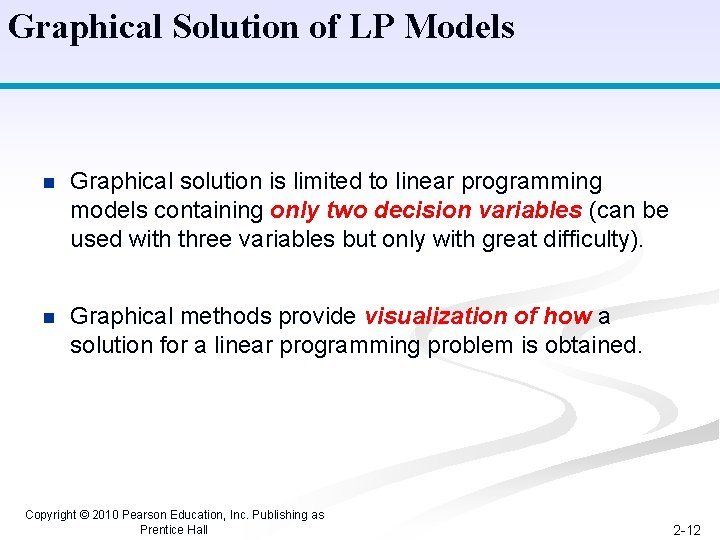Graphical Solution of LP Models n Graphical solution is limited to linear programming models containing only two decision variables (can be used with three variables but only with great difficulty). n Graphical methods provide visualization of how a solution for a linear programming problem is obtained. Copyright © 2010 Pearson Education, Inc. Publishing as Prentice Hall 2 -12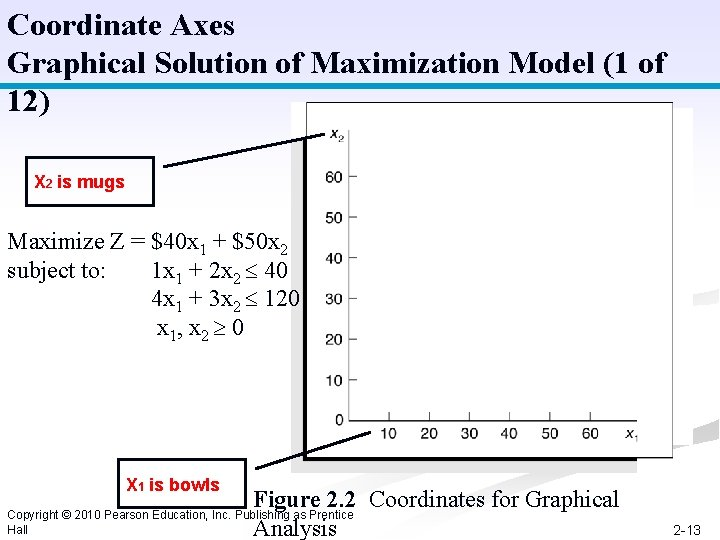Coordinate Axes Graphical Solution of Maximization Model (1 of 12) X 2 is mugs Maximize Z = \$40 x 1 + \$50 x 2 subject to: 1 x 1 + 2 x 2 40 4 x 1 + 3 x 2 120 x 1, x 2 0 X 1 is bowls Figure 2. 2 Coordinates for Graphical Analysis Copyright © 2010 Pearson Education, Inc. Publishing as Prentice Hall 2 -13Labor Constraint Graphical Solution of Maximization Model (2 of 12) Maximize Z = \$40 x 1 + \$50 x 2 subject to: 1 x 1 + 2 x 2 40 4 x 1 + 3 x 2 120 x 1, x 2 0 Figure 2. 3 Graph of Labor Constraint Copyright © 2010 Pearson Education, Inc. Publishing as Prentice Hall 2 -14Labor Constraint Area Graphical Solution of Maximization Model (3 of 12) Maximize Z = \$40 x 1 + \$50 x 2 subject to: 1 x 1 + 2 x 2 40 4 x 1 + 3 x 2 120 x 1, x 2 0 Figure 2. 4 Labor Constraint Area Copyright © 2010 Pearson Education, Inc. Publishing as Prentice Hall 2 -15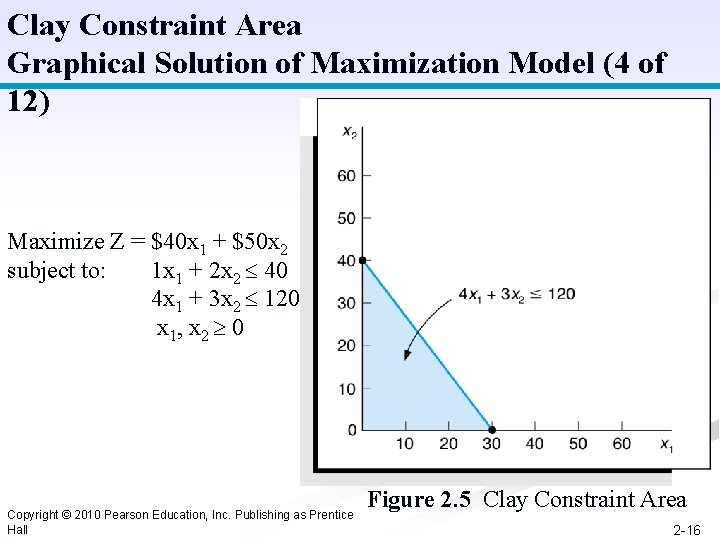Clay Constraint Area Graphical Solution of Maximization Model (4 of 12) Maximize Z = \$40 x 1 + \$50 x 2 subject to: 1 x 1 + 2 x 2 40 4 x 1 + 3 x 2 120 x 1, x 2 0 Copyright © 2010 Pearson Education, Inc. Publishing as Prentice Hall Figure 2. 5 Clay Constraint Area 2 -16Both Constraints Graphical Solution of Maximization Model (5 of 12) Maximize Z = \$40 x 1 + \$50 x 2 subject to: 1 x 1 + 2 x 2 40 4 x 1 + 3 x 2 120 x 1, x 2 0 Figure 2. 6 Graph of Both Model Constraints Copyright © 2010 Pearson Education, Inc. Publishing as Prentice Hall 2 -17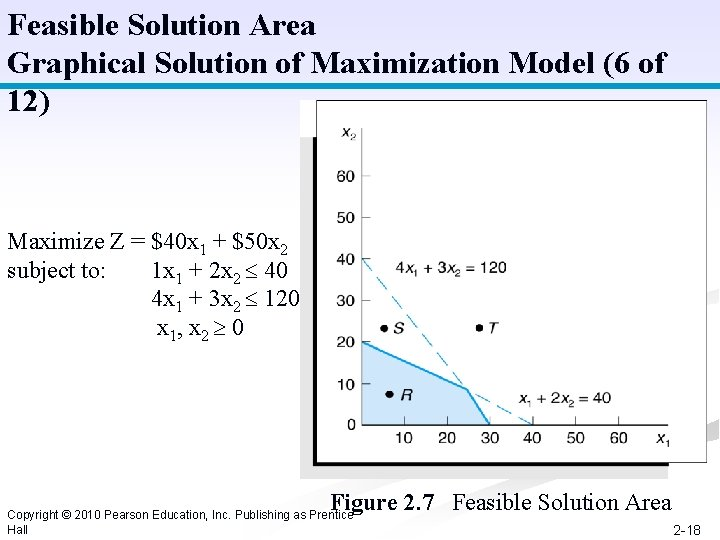Feasible Solution Area Graphical Solution of Maximization Model (6 of 12) Maximize Z = \$40 x 1 + \$50 x 2 subject to: 1 x 1 + 2 x 2 40 4 x 1 + 3 x 2 120 x 1, x 2 0 Figure 2. 7 Feasible Solution Area Copyright © 2010 Pearson Education, Inc. Publishing as Prentice Hall 2 -18Objective Function Solution = \$800 Graphical Solution of Maximization Model (7 of 12) Maximize Z = \$40 x 1 + \$50 x 2 subject to: 1 x 1 + 2 x 2 40 4 x 1 + 3 x 2 120 x 1, x 2 0 Figure 2. 8 Objection Function Line for Z = \$800 Copyright © 2010 Pearson Education, Inc. Publishing as Prentice Hall 2 -19Alternative Objective Function Solution Lines Graphical Solution of Maximization Model (8 of 12) Maximize Z = \$40 x 1 + \$50 x 2 subject to: 1 x 1 + 2 x 2 40 4 x 1 + 3 x 2 120 x 1, x 2 0 Figure 2. 9 Alternative Objective Function Lines Copyright © 2010 Pearson Education, Inc. Publishing as Prentice Hall 2 -20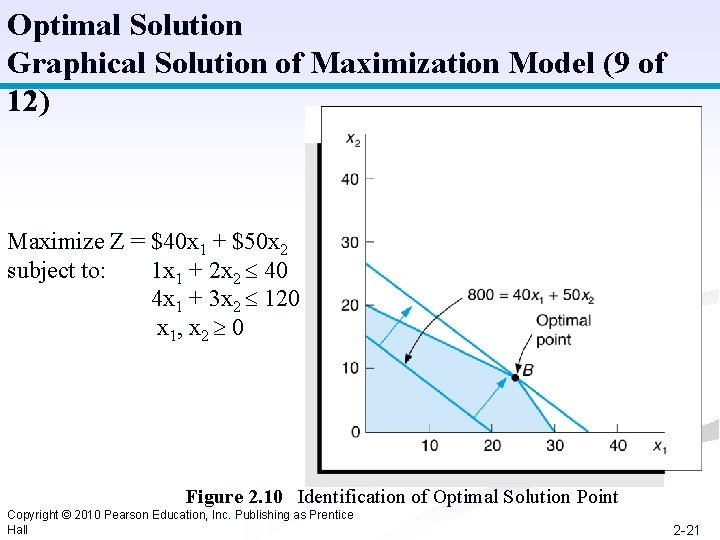Optimal Solution Graphical Solution of Maximization Model (9 of 12) Maximize Z = \$40 x 1 + \$50 x 2 subject to: 1 x 1 + 2 x 2 40 4 x 1 + 3 x 2 120 x 1, x 2 0 Figure 2. 10 Identification of Optimal Solution Point Copyright © 2010 Pearson Education, Inc. Publishing as Prentice Hall 2 -21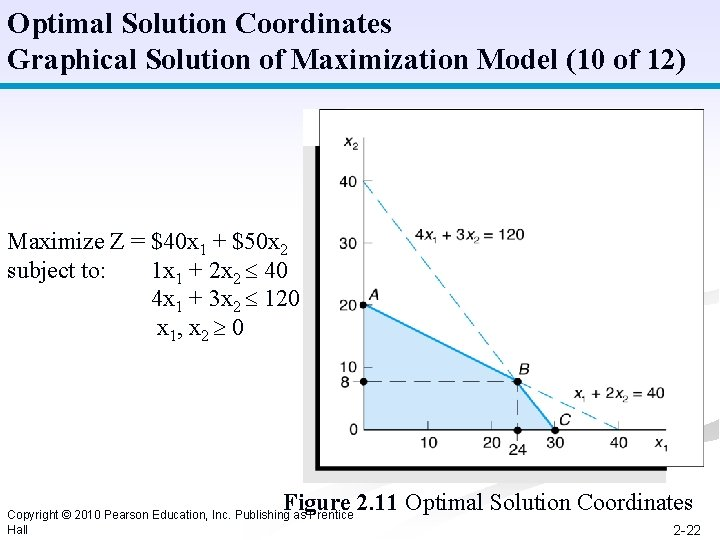Optimal Solution Coordinates Graphical Solution of Maximization Model (10 of 12) Maximize Z = \$40 x 1 + \$50 x 2 subject to: 1 x 1 + 2 x 2 40 4 x 1 + 3 x 2 120 x 1, x 2 0 Figure 2. 11 Optimal Solution Coordinates Copyright © 2010 Pearson Education, Inc. Publishing as Prentice Hall 2 -22Extreme (Corner) Point Solutions Graphical Solution of Maximization Model (11 of 12) Maximize Z = \$40 x 1 + \$50 x 2 subject to: 1 x 1 + 2 x 2 40 4 x 1 + 3 x 2 120 x 1, x 2 0 Figure 2. 12 Solutions at All Corner Points Copyright © 2010 Pearson Education, Inc. Publishing as Prentice Hall 2 -23Optimal Solution for New Objective Function Graphical Solution of Maximization Model (12 of 12) Maximize Z = \$70 x 1 + \$20 x 2 subject to: 1 x 1 + 2 x 2 40 4 x 1 + 3 x 2 120 x 1, x 2 0 Figure 2. 13 Optimal Solution with Z = 70 x 1 + 20 x 2 Copyright © 2010 Pearson Education, Inc. Publishing as Prentice Hall 2 -24Slack Variables n n Standard form requires that all constraints be in the form of equations (equalities). A slack variable is added to a constraint (weak inequality) to convert it to an equation (=). n A slack variable typically represents an unused resource. n A slack variable contributes nothing to the objective function value. Copyright © 2010 Pearson Education, Inc. Publishing as Prentice Hall 2 -25Linear Programming Model: Standard Form Max Z = 40 x 1 + 50 x 2 + s 1 + s 2 subject to: 1 x 1 + 2 x 2 + s 1 = 40 4 x 1 + 3 x 2 + s 2 = 120 x 1, x 2, s 1, s 2 0 Where: x 1 = number of bowls x 2 = number of mugs s 1, s 2 are slack variables Figure 2. 14 Solution Points A, B, and C with Slack Copyright © 2010 Pearson Education, Inc. Publishing as Prentice Hall 2 -26